# For following two production functions: (1) Q = L1/4 K (2) Q = 2L + 4K...

For following two production functions: (1) Q = L1/4 K (2) Q = 2L + 4K a) Determine whether factor L is subject to diminishing marginal returns? What about K? b) Calculate the MRTS. c) Determine returns to scale: CRS, IRS or DRS. Show how you arrive at your answers. For function (2) also draw a few representative isoquants; fully label your graph.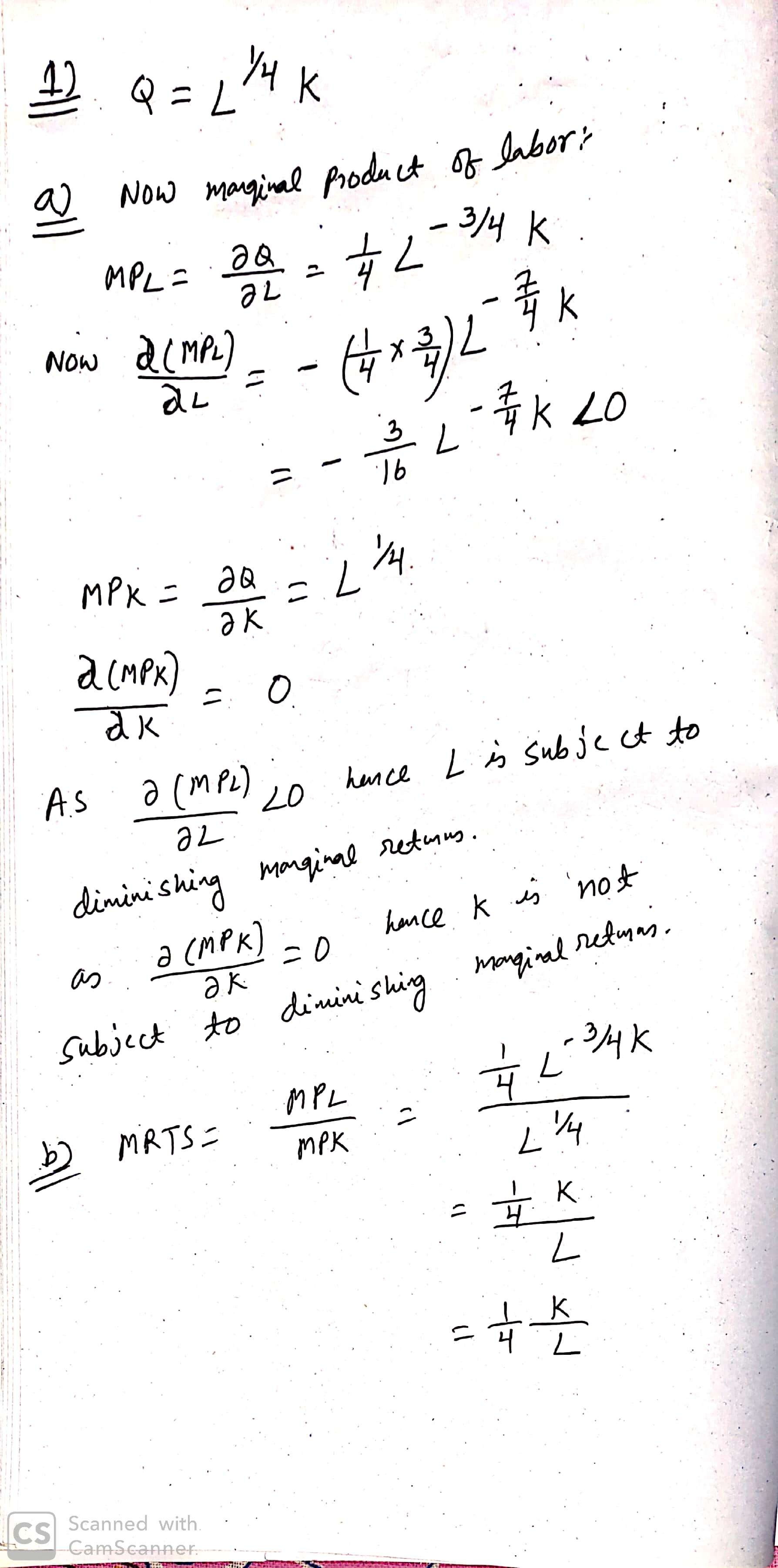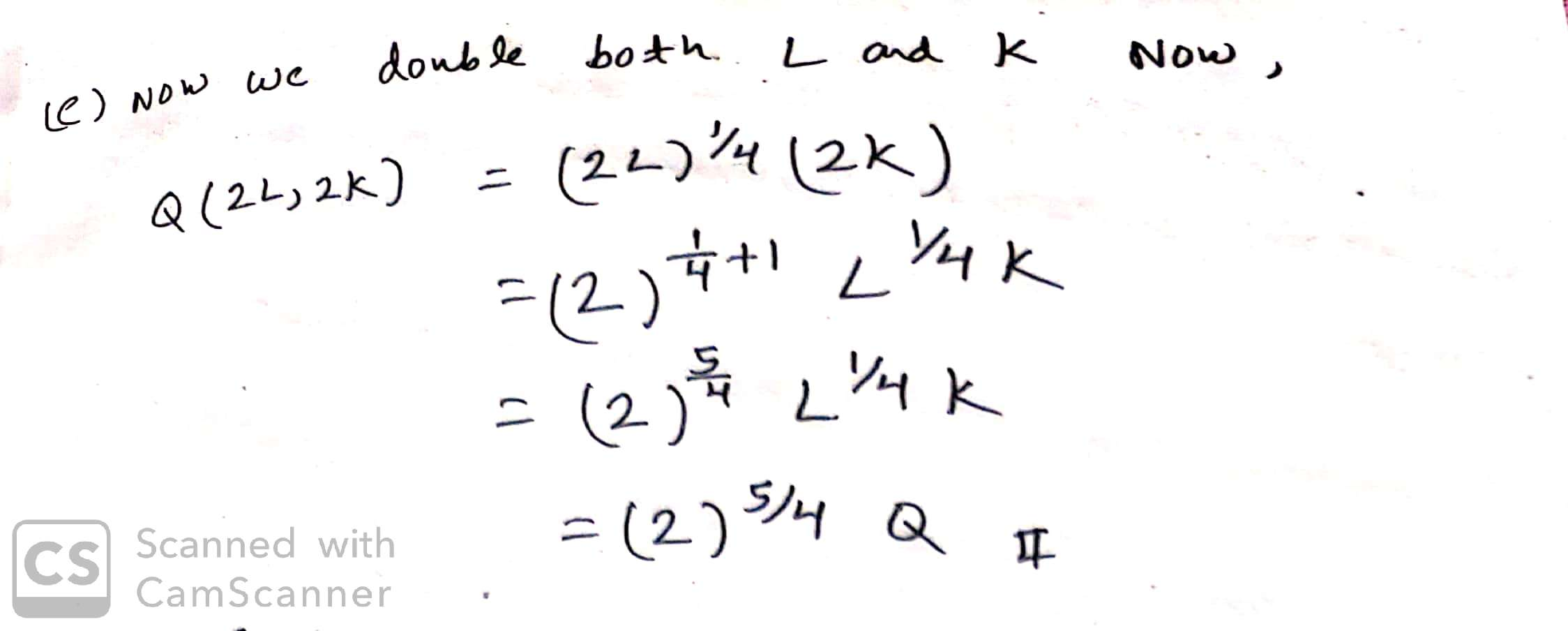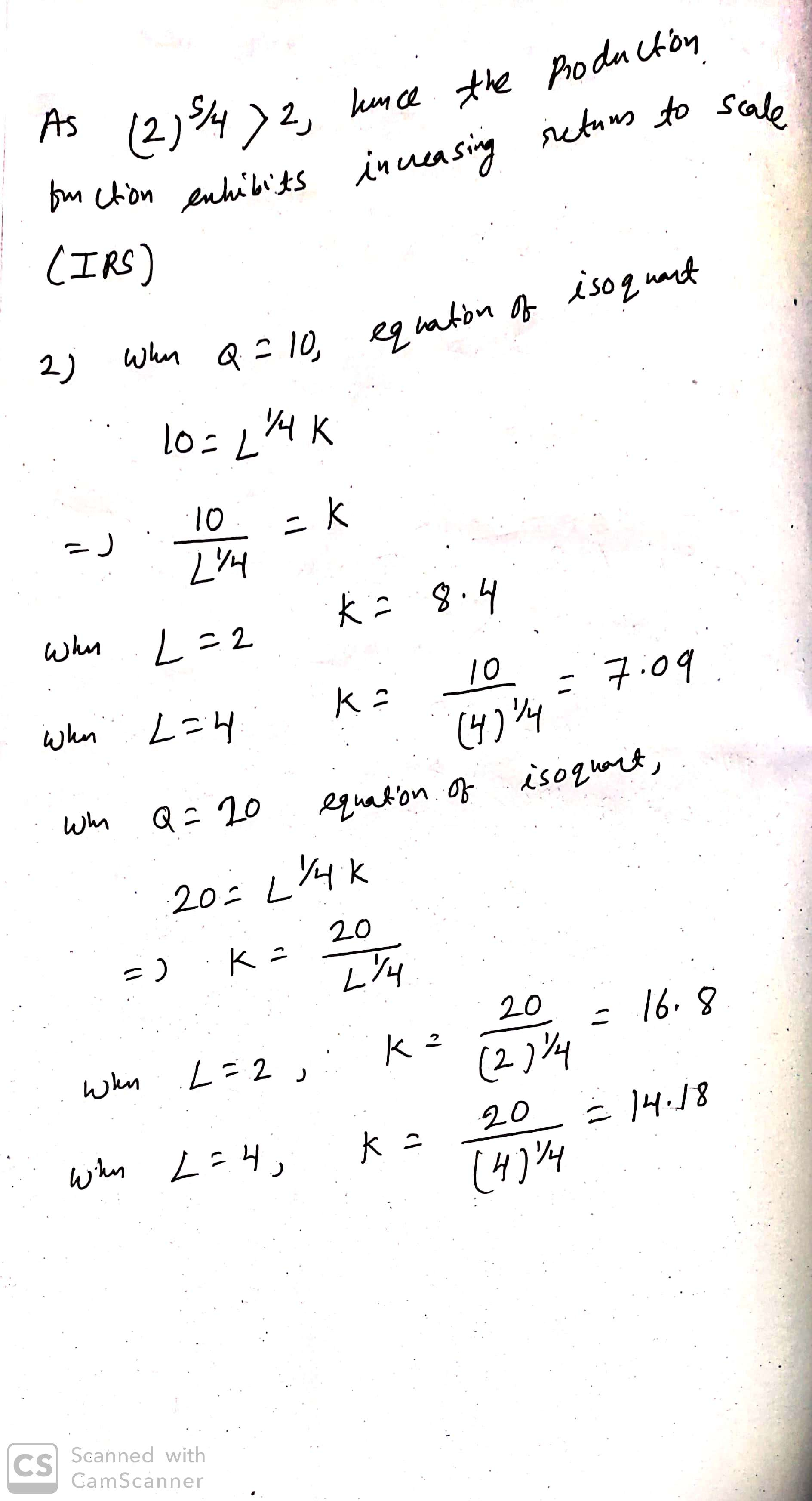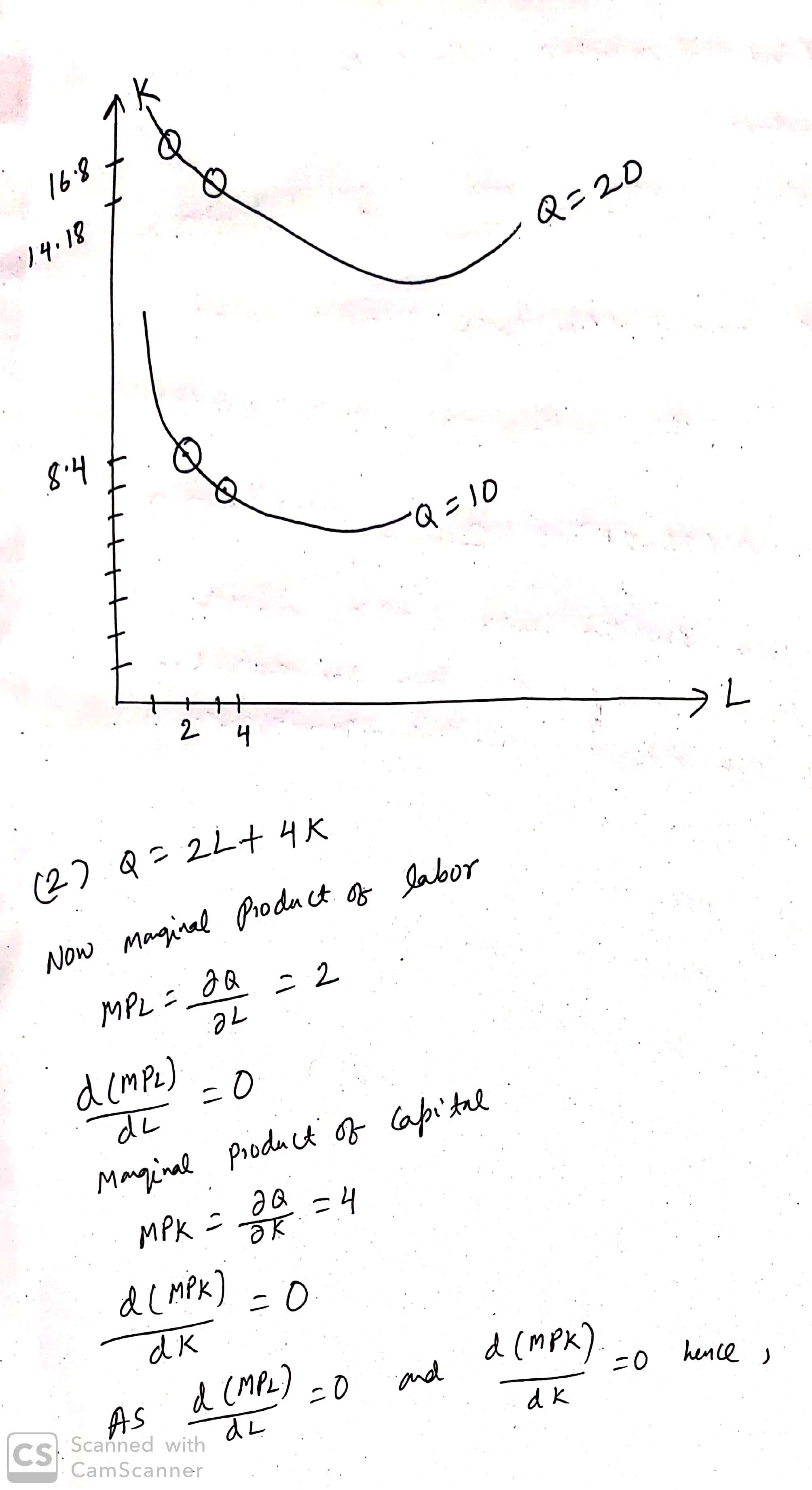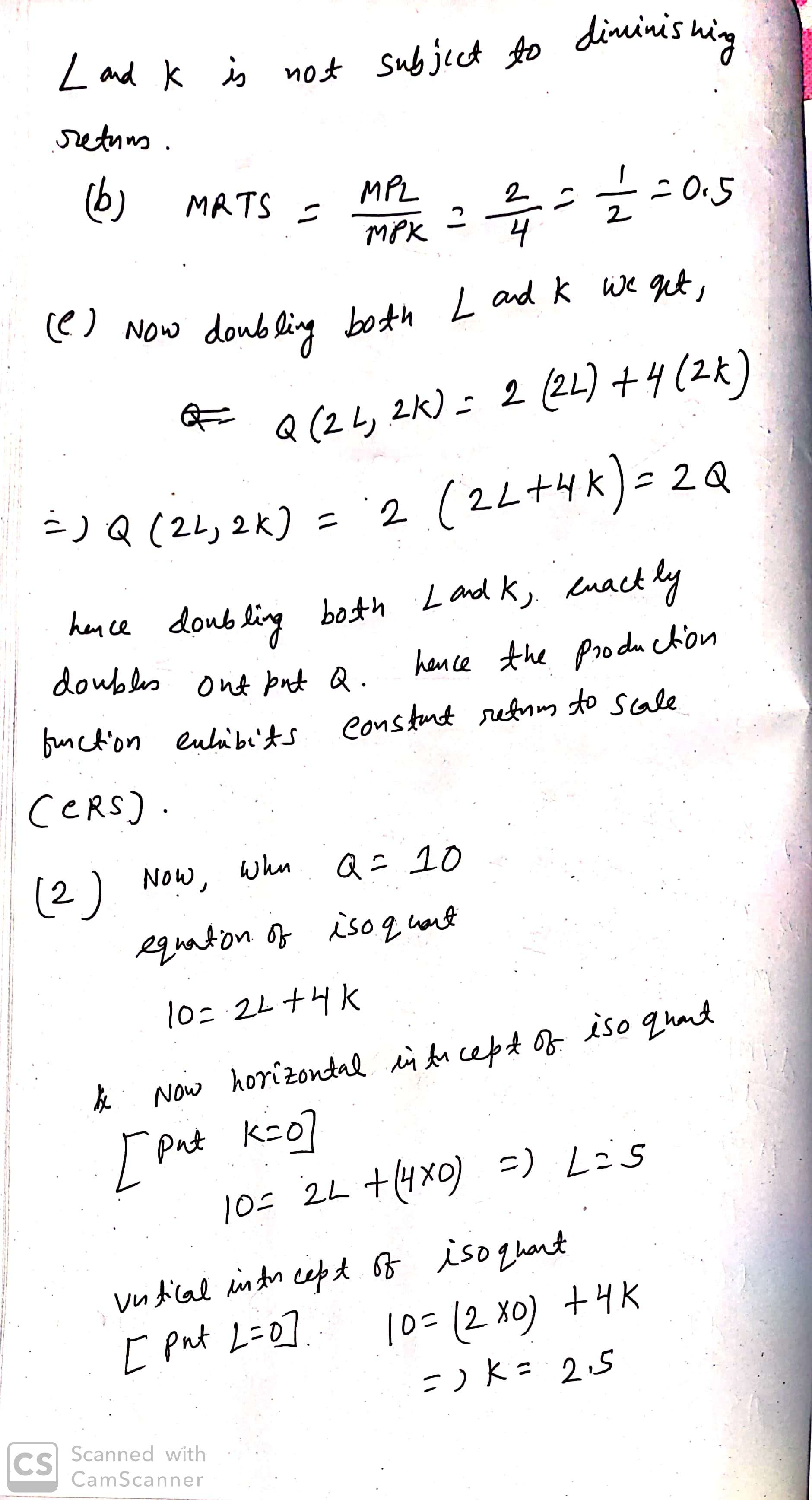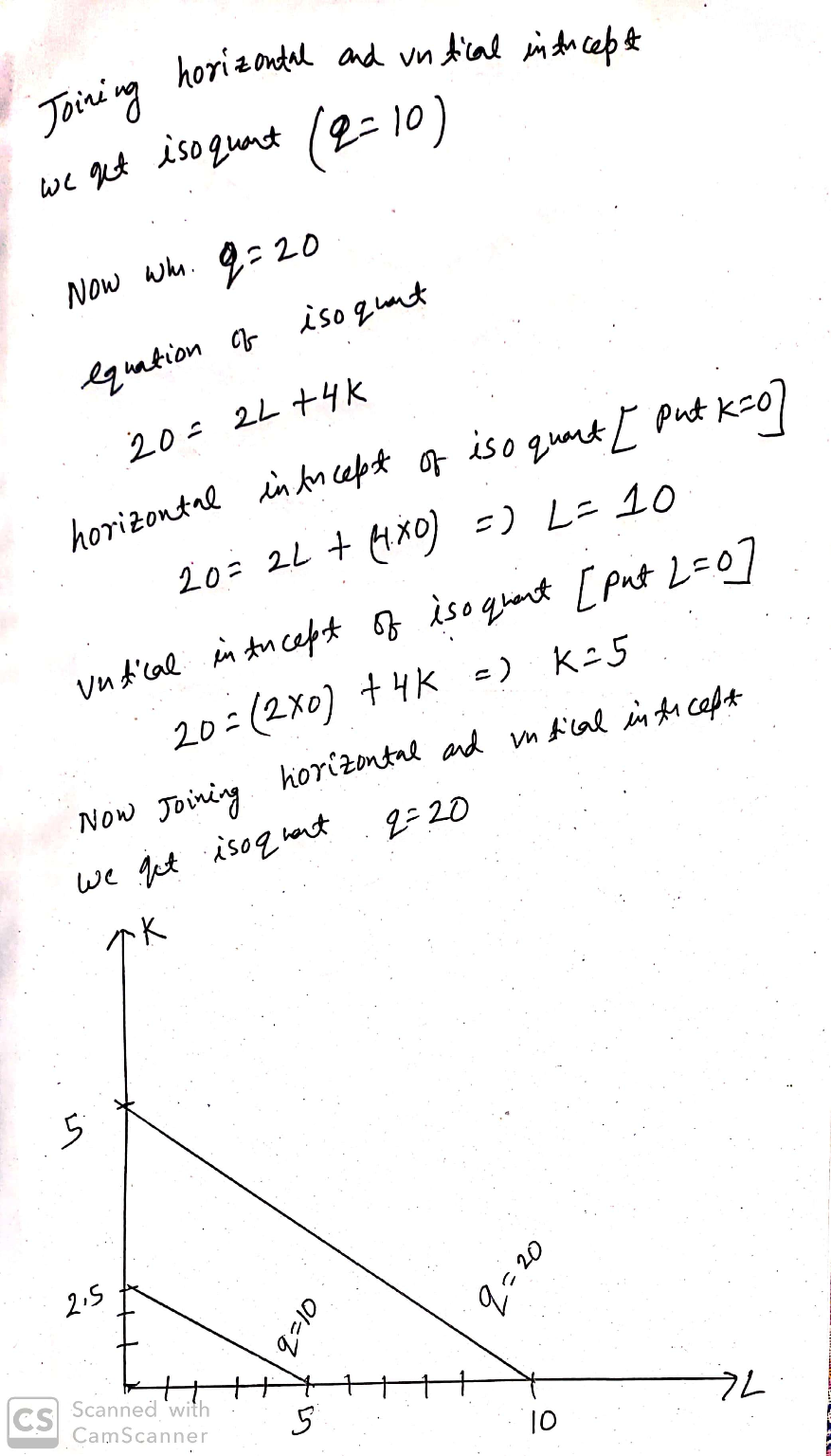#### Earn Coin

Coins can be redeemed for fabulous gifts.

Similar Homework Help Questions
• ### 1. Consider a firm that has the following CES production function: Q = f(L,K) = [aLP...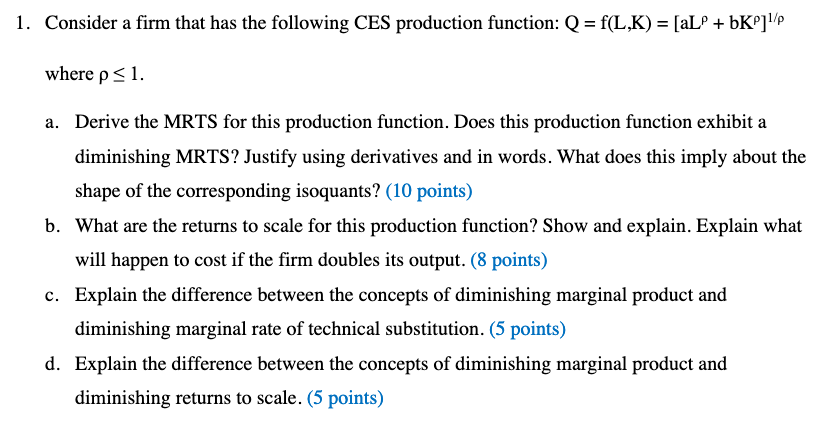1. Consider a firm that has the following CES production function: Q = f(L,K) = [aLP + bK°]!/p where p a. Derive the MRTS for this production function. Does this production function exhibit a diminishing MRTS? Justify using derivatives and in words. What does this imply about the shape of the corresponding isoquants? (10 points) b. What are the returns to scale for this production function? Show and explain. Explain what will happen to cost if the firm doubles its...

• ### Given the following long run production and cost functions: q=LPK1/4 C = 12L +4K (A) What...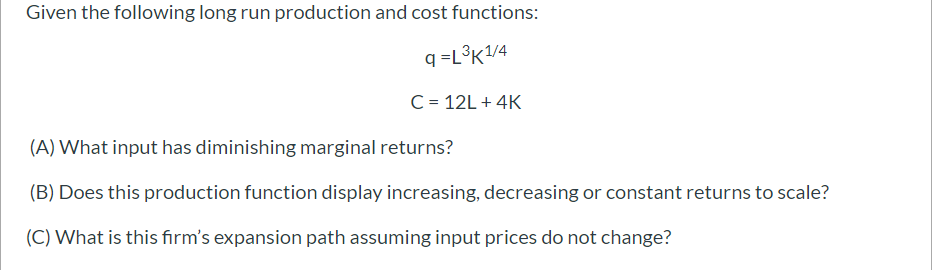Given the following long run production and cost functions: q=LPK1/4 C = 12L +4K (A) What input has diminishing marginal returns? (B) Does this production function display increasing, decreasing or constant returns to scale? (C) What is this firm's expansion path assuming input prices do not change? Clearly type out your answer to parts (A), (B) and (C) in the space provided. Retain all of your handwritten work for this question to be uploaded separately after you have completed the...

• ### Consider a firm that has the following CES production function: Q = f(L,K) = [aL^ρ + bK^ρ]^1/ρ where ρ ≤ 1. Please...

Consider a firm that has the following CES production function: Q = f(L,K) = [aL^ρ + bK^ρ]^1/ρ where ρ ≤ 1. Please clearly show each STEP and make sure your handwriting is LEGABLE. Thank you Derive the MRTS for this production function. Does this production function exhibit a diminishing MRTS? Justify using derivatives and in words. What does this imply about the shape of the corresponding isoquants? (10 points) What are the returns to scale for this production function? Show...

• ### 4. Consider the production functions given below: a. Suppose that the production function faced by a...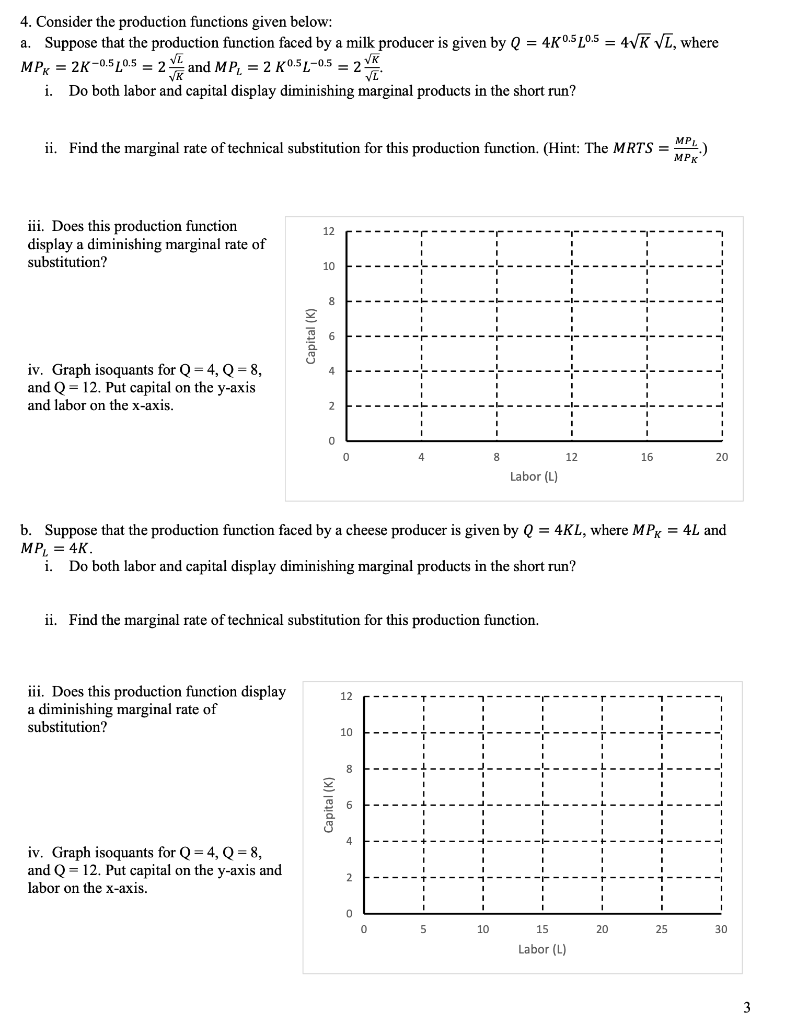4. Consider the production functions given below: a. Suppose that the production function faced by a milk producer is given by Q = 40.5 20.5 = 4VK VL, where MPx = 2K-0.5 20.5 = 2 and MP, = 2 K0.5L-05 = 2 * i. Do both labor and capital display diminishing marginal products in the short run? ii. Find the marginal rate of technical substitution for this production function. (Hint: The MRTS = 1) iii. Does this production function display...

• ### Given the following long run production and cost functions: q=L3K1/4 C = 12L +4K (A) What...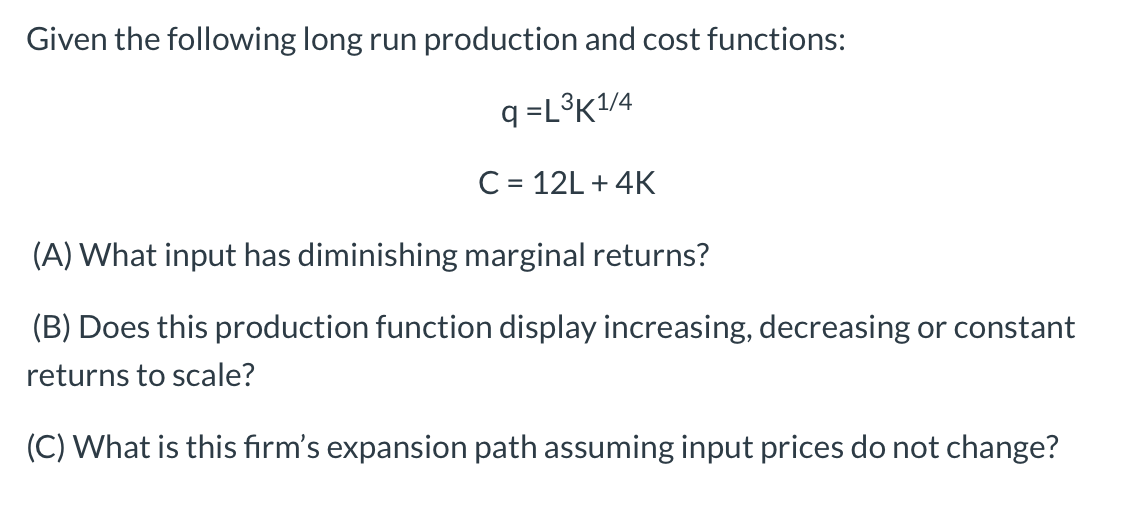Given the following long run production and cost functions: q=L3K1/4 C = 12L +4K (A) What input has diminishing marginal returns? (B) Does this production function display increasing, decreasing or constant returns to scale? (C) What is this firm's expansion path assuming input prices do not change?

• ### 3. For each of the following production functions, graph a typical isoquant and determine whether the marginal rate of...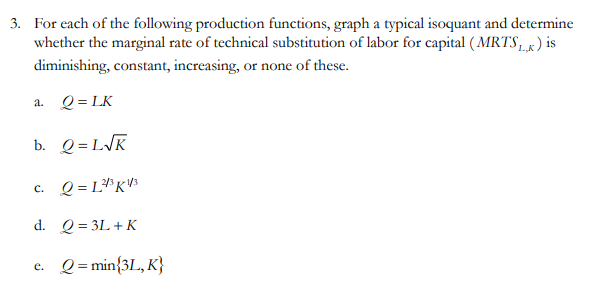3. For each of the following production functions, graph a typical isoquant and determine whether the marginal rate of technical substitution of labor for capital (MRTS ) is diminishing, constant, increasing, or none of these. a. Q=LK b. Q=LVK c. Q=L*K13 d. Q = 3L +K e. Q = min{3L, K} Show transcribed image text 3. For each of the following production functions, graph a typical isoquant and determine whether the marginal rate of technical substitution of labor for capital...

• ### 2. For each of the following production functions: Q = 3L2 +6K4 Q= V25KL Q =...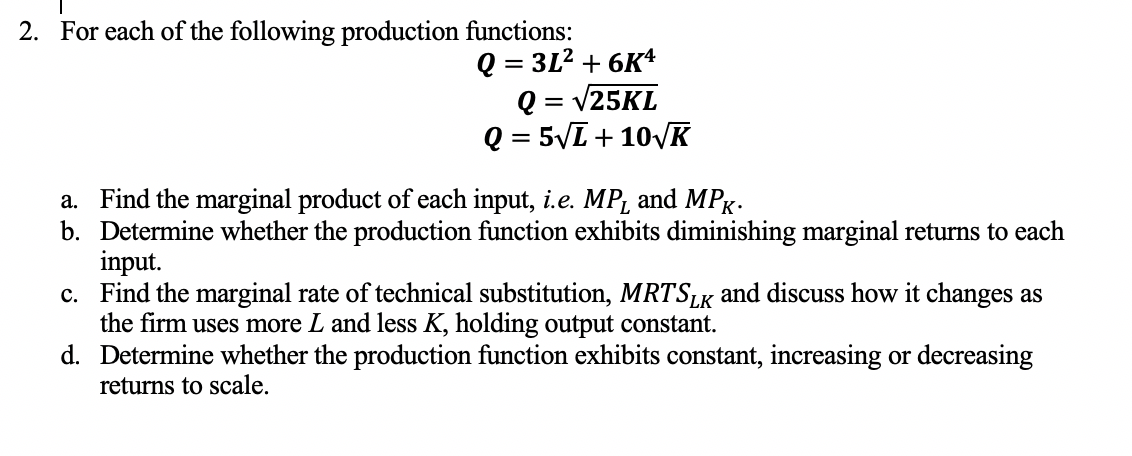2. For each of the following production functions: Q = 3L2 +6K4 Q= V25KL Q = 5V1 + 101K a. Find the marginal product of each input, i.e. MP, and MPx: b. Determine whether the production function exhibits diminishing marginal returns to each input. c. Find the marginal rate of technical substitution, MRTSLK and discuss how it changes as the firm uses more L and less K, holding output constant. d. Determine whether the production function exhibits constant, increasing or...

• ### 4. Below are production functions that turn capital (K) and labor (L) into output. For each...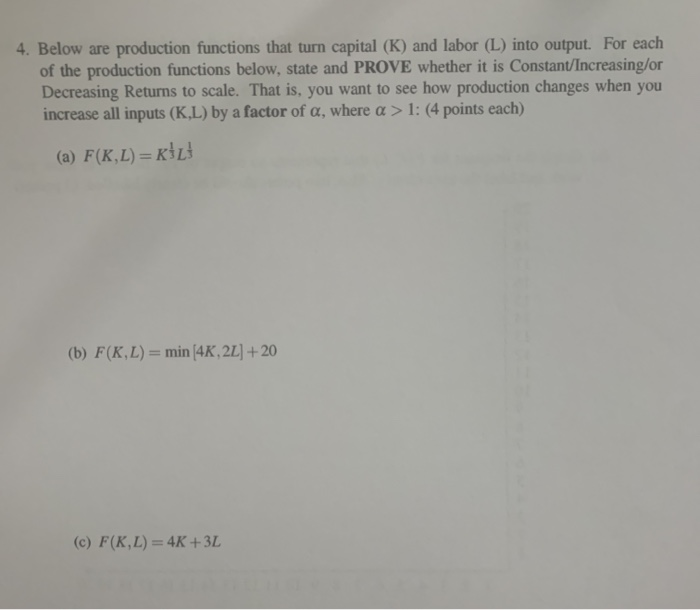4. Below are production functions that turn capital (K) and labor (L) into output. For each of the production functions below, state and PROVE whether it is Constant/Increasing/or Decreasing Returns to scale. That is, you want to see how production changes when you increase all inputs (K.L) by a factor of a, where a > 1: (4 points each) (a) F(K,L) =KİL (b) F(K,L) = min 4K, 2L] + 20 (c) F(K,L) = 4K +3L 5. For this problem you...

• ### Course Materials - 202001-12752 https://bb. 1. A firm has the following production function: 4 = 2L"2114...Course Materials - 202001-12752 https://bb. 1. A firm has the following production function: 4 = 2L"2114 a. Suppose K= l in the short run but L is variable. Let w denote the wage rate and letr denote the rental rate of capital. Solve for the firm's short run production function and short run cost function. (3 points). b. What is the marginal cost of an additional unit of g in the short run? Is it increasing, decreasing, or constant in...

• ### Consider a firm that faces the following production function: q = f(L, K) = L1/2 K1/2...

Consider a firm that faces the following production function: q = f(L, K) = L1/2 K1/2 where q is output, L is labor, and K is capital. Use this production function to answer the following questions. (a) What is the marginal product of labor (MPL)? (b) Does the MPL follow the law of diminishing returns? How do you know? (c) What is the marginal product of capital (MPK)? (d) Does the MPK follow the law of diminishing returns? How do...

Free Homework App